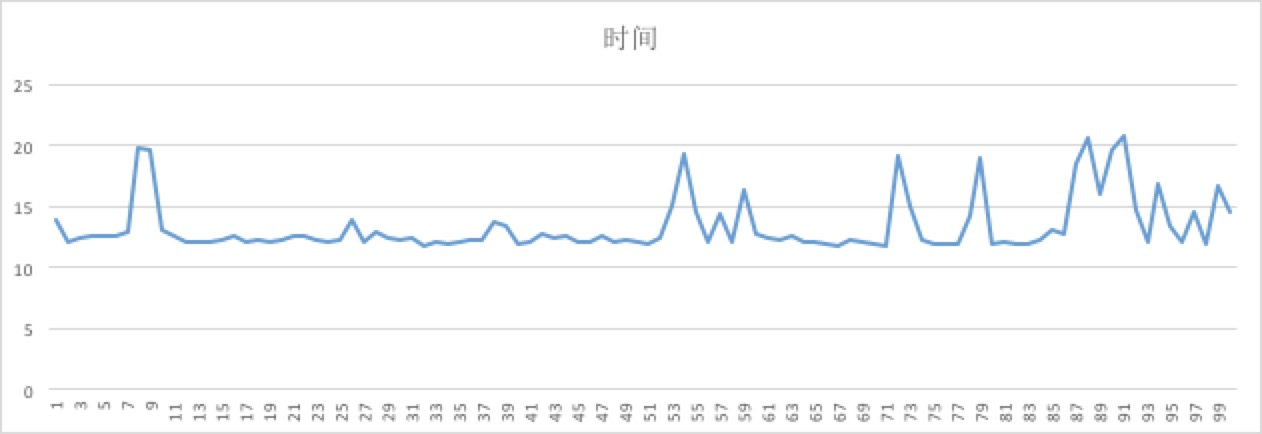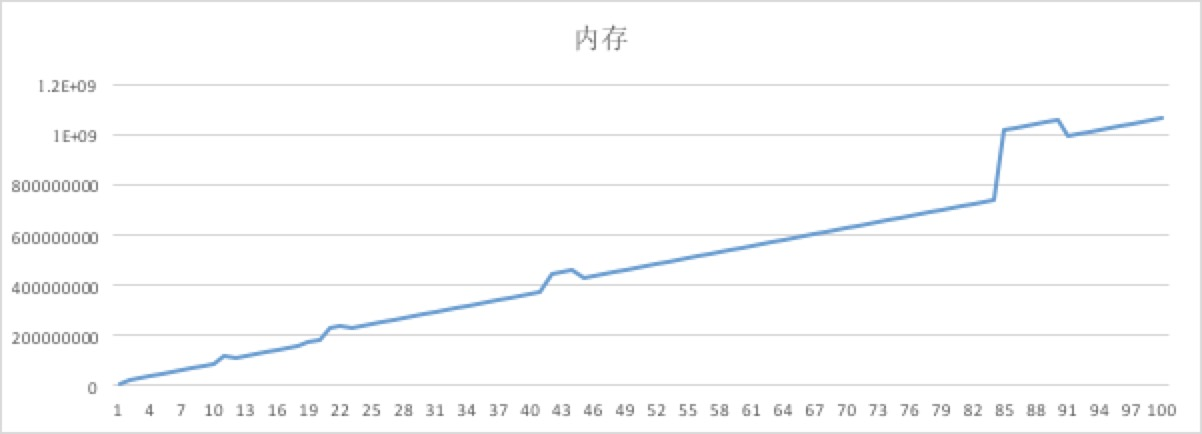# Redis 关于大量1级key的测试

版权声明 本站原创文章 由 萌叔 发表



## 1. 测试验证

### 1.1 测试方法

import json
import redis
import random
import time
import hashlib
r = redis.Redis(host='localhost',port=6379,db=5)

SIZE = 100000
fp = open('result.txt', 'w')
counter = 0
for i in xrange(0, 100):
t1 = time.time()
for j in xrange(0, SIZE):
counter += 1
print 'counter', counter
m = hashlib.md5()
m.update(str(i * SIZE + j))
key = m.hexdigest()
r.set(key, 1)
t2 = time.time()
margin = t2 - t1
info = r.info()
#print info
ll = []
ll.append( str((i + 1) * SIZE)  )
ll.append(str(margin))
ll.append(str(info['used_memory']))
fp.write(','.join(ll) + '\n')
fp.flush()
print (i + 1) * SIZE, margin, info['used_memory']

fp.close()


### 1.2 测试数据